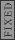GR 8677927796770177 | # Login | Register

GR9277 #79
Problem
 GREPhysics.NET Official Solution Alternate Solutions
\prob{79}The dispersion curve shown above relates the angular frequency to the wave number k. For waves with wave numbers lying in the range $k_1 1. They are in opposite directions. 2. They are in the same direction and the phase velocity is larger 3. They are in the same direction and the group velocity is larger 4. The phase velocity is infinite and the group velocity is finite. 5. They are the same in direction and magnitude Wave Phenomena}Group Velocity Recall that the group velocity is given by and the phase velocity is given by . In the region between and , the derivative is a constant negative quantity (approximately just the derivative of a line with negative slope). However, is positive in this region. Thus, the phase and group velocity are traveling in opposite directions. Thus, choose choice (A).Alternate Solutions  calcuttj2014-09-05 06:30:22 You can instantly eliminate D. If you don't remember specifically which is /k and which is /k, just remember that one depends on the derivative which is negative and the other is w/k which is positive and you can come to A. Reply to this commentComments calcuttj 2014-09-05 06:30:22 You can instantly eliminate D. If you don't remember specifically which is /k and which is /k, just remember that one depends on the derivative which is negative and the other is w/k which is positive and you can come to A.hoyas08 2007-12-30 12:08:56 The second sentence of the question doesn't display right for me. It should read: For waves with wave numbers lying in the range k₁ < k < k₂ , which of the following is true of the phase velocity and the group velocity?jcain6 2005-11-23 06:46:20 Choice (c) states that they are in the same direction and the group velocity is larger. This isn't true and just looks to be a typo on your part. Your description is consistent with choice (A) which is the correct answer.  yosun2005-11-23 14:59:43 jcain6: thanks for the typo-alert; it has been corrected.Post A Comment!  Username: Password: Click here to register. This comment is best classified as a: (mouseover)Mouseover the respective type above for an explanation of each type. ## Bare Basic LaTeX Rosetta Stone LaTeX syntax supported through dollar sign wrappers$, ex., $\alpha^2_0$ produces .
type this... to get...
$\int_0^\infty$
$\partial$
$\Rightarrow$
$\ddot{x},\dot{x}$
$\sqrt{z}$
$\langle my \rangle$
$\left( abacadabra \right)_{me}$
$\vec{E}$
$\frac{a}{b}$

The Sidebar Chatbox...
Scroll to see it, or resize your browser to ignore it...